# 35 Basic Pure Math Questions

The following is a list of basic pure maths questions that students should be able to tackle if they are prepared for a subset of the AS-Level Pure Maths exam. They have been designed to cover the techniques that will be needed in order to tackle some aspects of the pure maths exam. However, these questions do not necessarily resemble the type of exam question students might be faced with, these questions should be used to practice those techniques needed. Having said that, early questions in the exam may look a lot like the questions you see here.

1. Simplify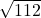.
2. Evaluate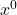.
3. Factorise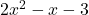.
4. Solve the inequality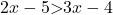.
5. Rationalise the denominator of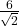.
6. How many roots does the graph of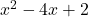have?
7. Evaluate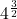.
8. Let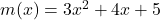. Find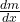and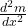.
9. Sketch the graph of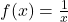.
10. Solve the simultaneous equations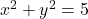and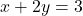.
11. The first term of a sequence is 58 and the common difference is -2. Find the 107th term.
12. Rationalise the denominator of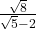.
13. Find the set of values of k for which the quadratic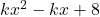has two different roots.
14. Integrate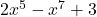.
15. Evaluate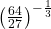.
16. Factorise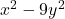.
17. Sketch the graph of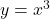.
18. The first 10 terms of a sequence, whose common difference is 4, adds up to 210. Find the first term.
19. Simplify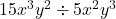.
20. Differentiate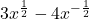.
21. Solve the inequality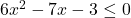.
22. Using the quadratic formula, solve the equation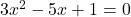. Leave your answer in surd form.
23. Sketch the graph of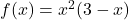.
24. If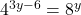, find the value of y.
25. Integrate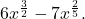26. Let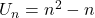. Find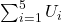.
27. Solve by completing the square: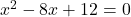28. Differentiate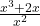.
29. Write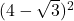in the form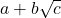.
30. Sketch the graph of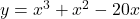.
31. Write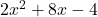in the form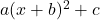.
32. Find the equation of the tangent to the curve of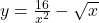when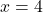.
33. The curve of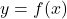such that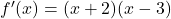and passes though the point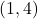. Find the equation for y.
34. A line passes through the points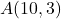and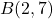. Find the midpoint of AB and the equation of the line that passes through A and B.
35. Sketch the graph of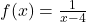.

Like us on FaceBook and register with us to keep up to date.